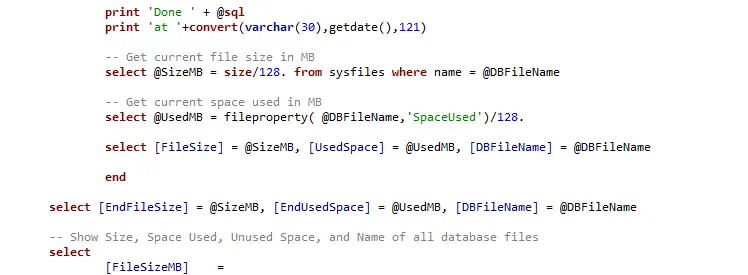# Why use WHERE 1=1 in SQL Queries

A common SQL question is, why would anyone use WHERE 1=1 in their queries? And what does it do?

The WHERE 1=1 condition means WHERE TRUE. It returns the same query result as it would without the WHERE Clause. There is no impact on query execution time.

This is something you can add to a SQL query for convenience for adding & replacing conditions on the statement. If you have WHERE 1=1 on a SQL query you are writing, all conditions thereafter will contain AND, so it’s easier when commenting out conditions on exploratory SQL queries.

## Example: WHERE 1 Equals 1

```/* where 1=1 example */
SELECT *
FROM TABLE
WHERE 1=1
--  and column1='blah'
and column2='more_blah'
--  and column3 is not null```

You can see this would be easier for commenting out WHERE conditions in the SQL ^

## Example (Extra): Commenting Columns

This is similar to another SQL querying technique where you have commas before column names, rather than after the column name.

Again, this might work out well for you when commenting out columns on a work-in-progress SQL query.

```SELECT
Column1
--  ,Column2
,Column3
,Column4
--  ,Column5
FROM TABLE```

Personally, I often make use of both of the above techniques when I write SQL queries.

Convenience is everything when working behind a computer. We have to keep exploring new short keys and any general ways of working to optimize our efficiency. Some things you might not like and won’t adopt, and sometimes GUI is better than CLI. It’s all personal preference, in the end, choose what you are most optimal with.

If you wonder what would happen if we change the WHERE 1=1 to WHERE 1=2, have a look at my other blog post – Why Use WHERE 1=2 in SQL

1.